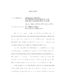## Minimal Surfaces, Hyperbolic 3-manifolds, and Related Deformation Spaces.Given a closed, oriented, smooth surface $Sigma$ of negative Euler characteristic, the relationships between three deformation spaces of geometric structures are compared: the space of minimal hyperbolic germs $mathcal{H},$ the space of representations $mathcal{R}(pi_1(Sigma),text{PSL}_2(mathbb{C})),$ and the space $mathcal{M}(X)$ of solutions to the self-duality equations on a rank-2 complex vector bundle over a Riemann surface $Xsimeq Sigma.$ Inside both $mathcal{H}$ and $mathcal{R}(pi_1(Sigma),text{PSL}_2(mathbb{C}))$ lies the space $mathcal{AF}$ of almost-Fuchsian manifolds comprised of quasi-Fuchsian 3-manifolds $Msimeq Sigma times mathbb{R}$ which contain an immersed closed minimal surface whose principal curvatures lie in the interval $(-1,1).$ The structure of these manifolds is explored through a study of the domain of discontinuity of the associated almost-Fuchsian holonomy group. It is proved that there are no doubly degenerate geometric limits of almost-Fuchsian manifolds. Next, the space $mathcal{H}$ is studied through an analysis of a smooth real valued function which records the topological entropy of the geodesic flow arising from a minimal hyperbolic germ. Estimates on this function are obtained which culminate in a new lower bound on the Hausdorff dimension of the limit set of a quasi-Fuchsian group. As a corollary we obtain a new proof of Bowen's theorem on quasi-circles: a quasi-Fuchsian group is Fuchsian if and only if the Hausdorff dimension of its limit set is equal to $1.$ Lastly, we recall a construction of Donaldson which shows how each minimal hyperbolic germ gives rise to a solution of the self-duality equations. In this context, we compare various deformations of a Fuchsian representation $pi_1(Sigma)rightarrow text{PSL}_2(mathbb{R}),$ finally obtaining an explicit formula for a deformation arising from minimal surfaces in terms of Fuchsian and bending deformations. Interestingly, the hyperk"{a}hler structure on the moduli space $mathcal{M}$ of solutions to the self-duality equations makes an appearance here.